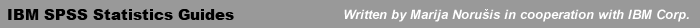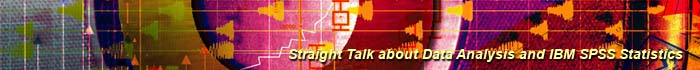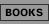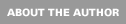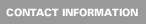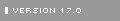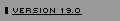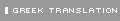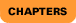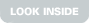IBM SPSS Statistics 19 Guide to Data Analysis: Chapters

### Part I. Getting Started with IBM SPSS Statistics

1. Introduction. The first chapter is a roadmap that discusses the goals and organization of the book.

2. An Introductory Tour of IBM SPSS Statistics. You learn to open data files, enter data into the data editor, and examine your results in the viewer window. Particular emphasis is on using the SPSS Statistics online tutorial and the online help system, so that you can always find the information that you need to run the software.

3. Sources of Data. What's important when designing a survey or an experiment? How should you ask a question? How should you select participants? How should you assign people to experimental groups? Why is it important to include a comparison group?

### Part II. Describing Data

4. Counting Responses. How can you summarize the various responses people give to a question? What is a frequency table and what can you learn from it? How can you tell from a frequency table if there have been errors in coding or entering data? What are percentages and cumulative percentages? When do you use pie charts, bar charts and histograms?

5. Computing Descriptive Statistics. How can you summarize the values of a variable? What are scales of measurement and why are they important? How does the arithmetic mean differ from the mode and the median? When is the median a better measure of central tendency than the mean? What does the variance tell you? The coefficient of variation? What are standardized scores, and why are they useful?

6. Comparing Groups. How can you determine if the values of the summary statistics for a variable differ for subgroups of cases? What are subgroups of cases? What can you learn from calculating summary statistics separately for subgroups of cases? How can you graph summary statistics for subgroups?

7. Looking at Distributions. What additional displays are useful for summarizing the distribution of a variable for several groups? What is a stem-and-leaf plot? How does a stem-and-leaf plot differ from a histogram? What is a boxplot?

8. Counting Responses for Combinations of Variables. How can you study the relationship between two or more variables that have a small number of possible values? Why is a frequency table not enough? What is a crosstabulation? What kind of percentages can you calculate for a crosstabulation and how do you choose among them? What is a dependent variable? An independent variable? How do you examine more than two variables together? How can you use a chart to display a crosstabulation?

9. Plotting Data. How can you display the relationship between two variables that are measured on a scale with meaningful numeric values? What is a scatterplot, and why is it useful? What is a scatterplot matrix? How do you identify unusual observations on a scatterplot? What can you learn from a three dimensional plot?

### Part III. Testing Hypotheses

10. Evaluating Results from Samples. What can you say about a population, based on the results you observe in a random sample? Are the results you observe in a sample identical to the results you would observe from the entire population? What is a sampling distribution? How is it used to test hypotheses about the population? What factors determine how much sample means vary from sample to sample? What is an observed significance level?

11. The Normal Distribution. What is the normal distribution, and why is it important for data analysis? What does a normal distribution look like? What is the standard normal distribution? What is the Central Limit Theorem, and why is it important?

12. Testing a Hypothesis about a Single Mean. How can you test the hypothesis that a sample comes from a population with a known mean? What are confidence intervals and why they are useful? What is a null hypothesis? An alternative hypothesis?

13. Testing a Hypothesis about Two Related Means. How can you test the hypothesis that the average difference between a pair of measurements is 0? What are paired experimental designs, and what are their advantages? What types of problems can occur when you use paired designs? What is a paired t-test? What are Type 1 and Type 2 errors? Why do you use a normal probability plot?

14. Testing a Hypothesis about Two Independent Means. How can you test whether two population means are equal, based on the results observed in two independent samples? Why can't you use a one-sample t test? What assumptions are needed? Can you prove that the null hypothesis is true? What is power, and why is it important?

15. One-Way Analysis of Variance. How can you test the null hypothesis that several population means are equal? What is analysis of variance? What assumptions about the data are needed to use analysis of variance? How is the F ratio computed, and what does it tell you? Why do you need multiple comparison procedures?

16. Two-Way Analysis of Variance. How can you test hypotheses about population means when you have two factors? What is a factor? What hypotheses can be tested? What is an interaction? What assumptions do you need? What problems do you encounter if you have unequal numbers of cases in the cells?

17. Comparing Observed and Expected Counts. How can you test the null hypothesis that two variables are independent? What are observed and expected counts? How do you compute the chi-square statistic? What assumptions are needed for the chi-square test of independence? When do you use a one sample chi-square test? Why is sample size important?

18. Nonparametric Tests. What are nonparametric tests, and when do you use them? When do you use the sign test? What is the Wilcoxon signed-rank test, and what is its advantage over the sign test? When do you use the Kruskal-Wallis test? Why would you use the runs test?

### Part IV. Examining Relationships

19. Measuring Association. How can you measure the strength of the relationship between two categorical variables? What are measures of association, and why are they useful? Is there a single best measure? Is the chi-square statistic a good measure of association? What is proportional reduction in error? When a measure of association is 0, does that always mean that the two variables are unrelated? How can additional information about order be incorporated into measures of association? How do you quantify agreement between two raters?

20. Linear Regression and Correlation. How can you choose the line that best summarizes the linear relationship between two variables? What is the least-squares line? What does the slope tell you? The intercept? How can you tell how well a line fits the data? How do you calculate predicted values and residuals?

21. Testing Regression Hypotheses. How can you test hypotheses about the population regression line based on the results you obtain in a sample? What is the population regression line? What assumptions do you have to make about the data to test hypotheses about the population regression line? How do you test hypotheses about the slope and the correlation coefficient? What is the difference between the confidence interval for the mean prediction and the prediction interval for an individual case?

22. Analyzing Residuals. How can you tell if the assumptions necessary for hypothesis testing in regression are violated? How do you use residuals to check the assumptions of independence, linearity, normality, and constant variance? What do you do if assumptions are violated? What are influential points and why they are important?

23. Building Multiple Regression Models. How do you build a regression model with more than one independent variable? What are partial regression coefficients? How can you test the null hypothesis that all of the population partial regression coefficients are 0? What can you tell from the partial regression coefficients about the relationship between the dependent variable and an independent variable? What are variable selection methods?

24. Multiple Regression Diagnostics. How can you check for violations of the multiple regression assumptions? Why are residual plots useful? How can you identify cases that are influencing the regression results more than the other cases are? What are leverage and Cook's distance? What can you learn from a partial regression plot?

### APPENDICES

A. Obtaining Charts in IBM SPSS Statistics.
B. Transforming and Selecting Data.
C. The T Distribution.
D. Areas under the Normal Curve.
E. Descriptions of Data Files.© 2005-2011 Marija Norušis: IBM SPSS Statistics Guides Site designed by Kenny Kim Photography